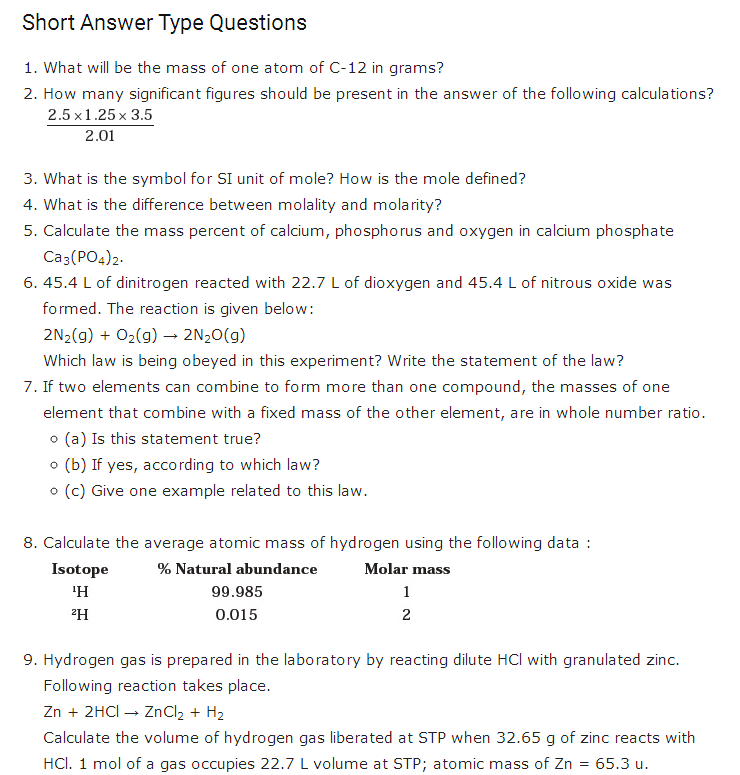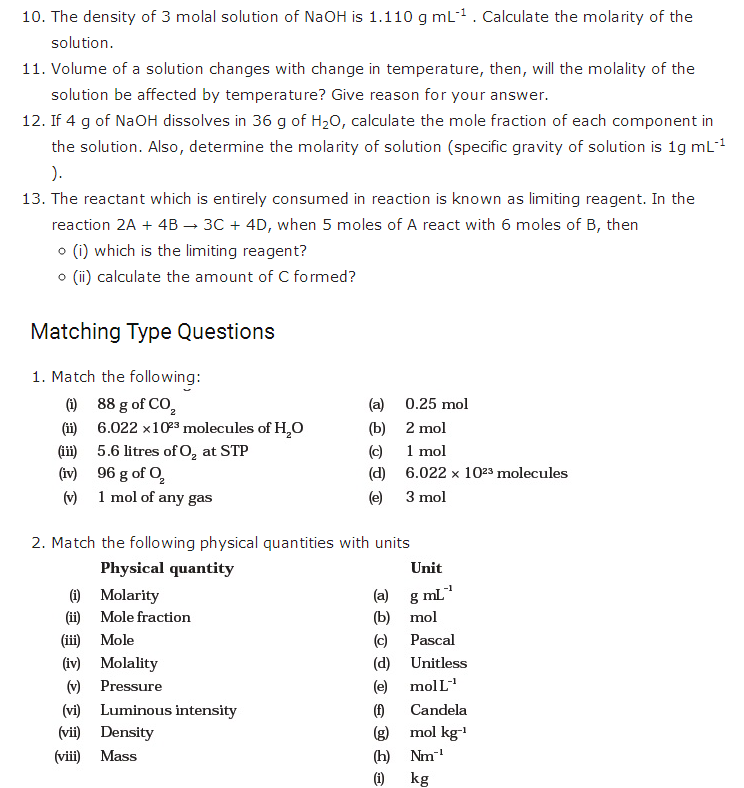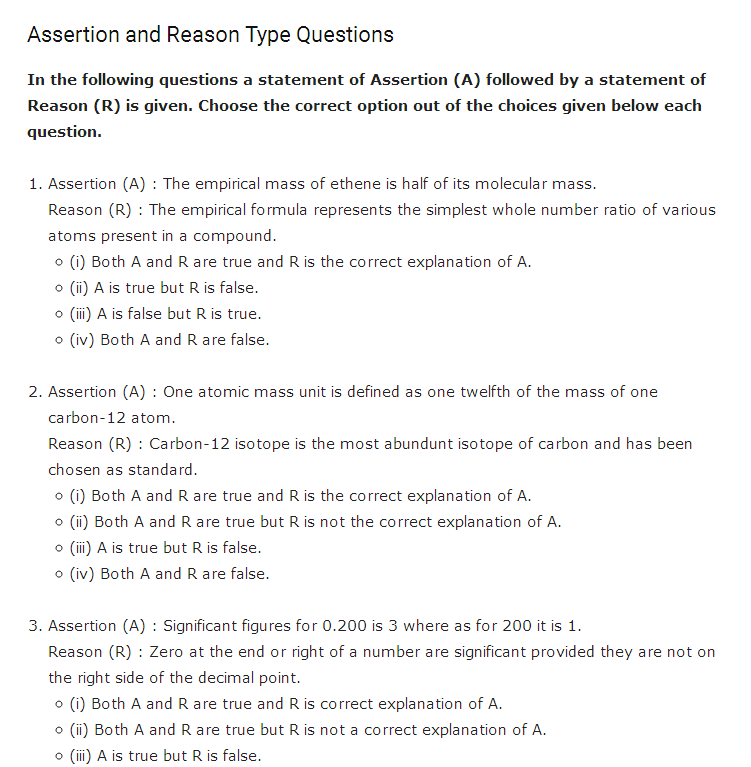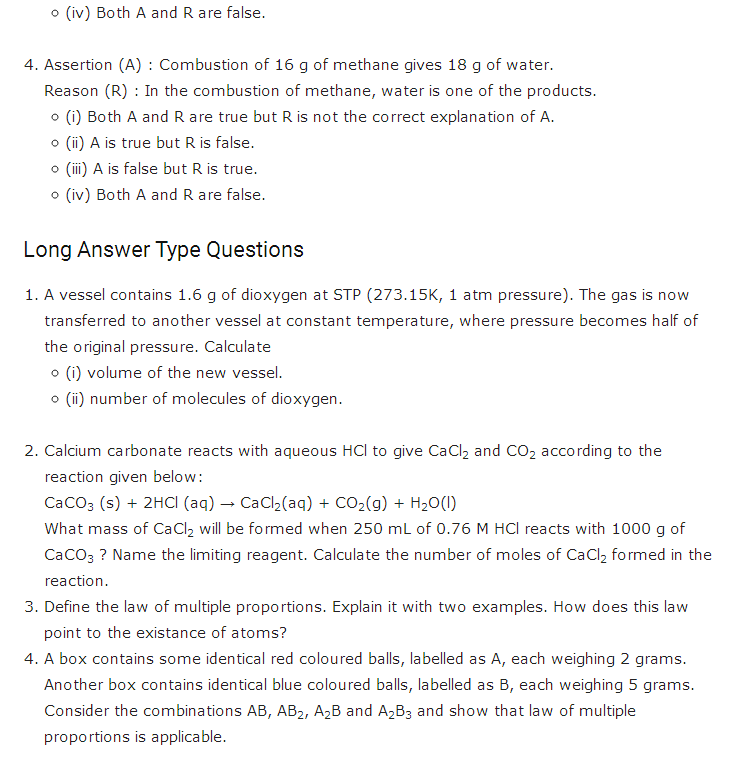# Chapter 1 - Some Basic Concepts of ChemistryAlso Access CBSE Class 11 chemistry Sample Papers

1. shankar singh

how to calculate the atomic mass of isotopes?

1. balaji

The atomic mass of isotopes are calculated by finding the no.of protons and neutrons and multiplying by 1amu

2. ayush

the sum of the numbers of protons and neutrons in the nucleus is called the mass number. This is because each proton and each neutron weigh one atomic mass unit (amu). By adding together the number of protons and neutrons and multiplying by 1 amu, you can calculate the mass of the atom.
it may help u

2. vijayasathya

very nice app

3. arbaz

nice

4. Naaz

❤️❤️excellent question bank❤️

5. folorunso mubarak

i love these group and i want to knw better